# How to use the NORM.S.DIST function in Excel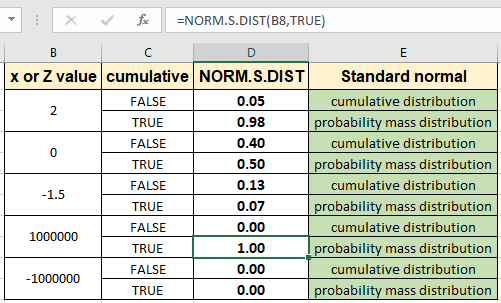In this article, we will learn How to use the NORM.S.DIST function in Excel.

What is Normal Cumulative Distribution?

Normal Cumulative Distribution is a type of continuous probability distribution for a real-valued random variable. The general form of its probability density function is given by :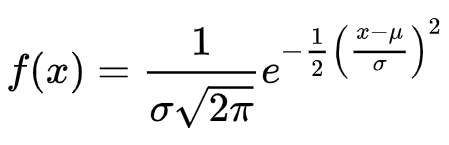The value is basically called the Z value. The given mathematical formula to calculate is very complex. So, You can calculate the normal cumulative distribution of the given specific mean and standard deviation for the dataset using the NORM.DIST Function. Calculate the inverse of normal cumulative distribution using the NORM.INV Function.

Standard Normal Cumulative Distribution is a special case of the normal cumulative distribution with mean () = 0  and Standard deviation () = 1. The general form of Standard Normal Cumulative Distribution is given by :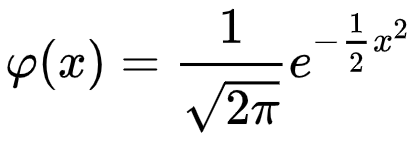You can calculate standard normal cumulative distribution using mean = 0 and standard deviation = 1 within NORM.DIST Function in Excel. The only variable the function relies on is the x value or else the z value.

Plot of Standard Normal distribution curve

The standard normal distribution has a mean of zero and a standard deviation of one. So, The probability density vs standard deviations curve for the standard normal cumulative distribution is shown below.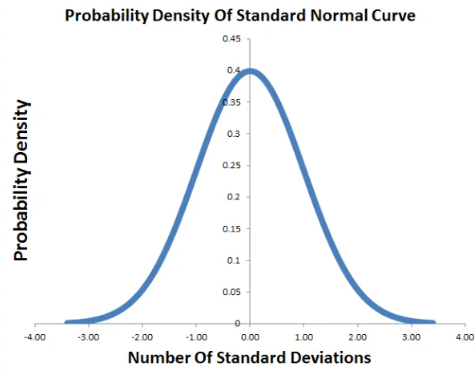The mathematical formula stated above is very complex to intrep formula wise, so excel gave the default formula. Now let's learn how to calculate the standard normal probability for cumulative distribution or probability mass distribution using the NORM.S.DIST function in Excel.

NORM.S.DIST function in Excel

The function does the same work as the mathematical formula. The function takes the x or z value as an argument and a cumulative argument which defines the type of result pdf or cdf. Lets understand more about arguments below

NORM.S.DIST Function syntax:

 =NORM.S.DIST(x,cumulative)

x :  value for which you want the distribution

cumulative : logical value that determines the form of the function. If cumulative is TRUE, NORM.S.DIST returns the cumulative distribution function; if FALSE, it returns the probability mass function.

Example :

All of these might be confusing to understand. Let's understand how to use the function using an example. Here we take some x values and learn the different distribution types. First we will evaluate the cumulative distribution having z value 2 for standard normal distribution which has default mean = 0 and standard deviation = 1

Use the formula:

 =NORM.S.DIST(B2, FALSE)

Or

 =NORM.S.DIST(B2, 0)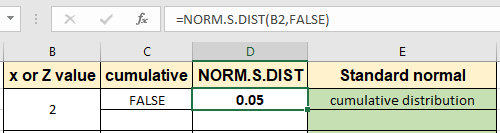The probability value corresponding to 2 comes out to be

0.05 for the standard normal distribution dataset. Now extract probability for the probability mass distribution for the same z value as shown below.

Use the formula:

 =NORM.S.DIST(B2, TRUE)

Or

 =NORM.S.DIST(B2, 1)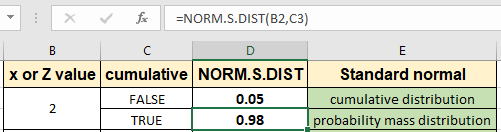As you can see the probability for this is much higher than the previous value. You can inspect the both values for the different z values. Just copy the formula to other cells using the Ctrl + D shortcut key or dragging down from the right bottom of the cell.As you can see the difference in results as shown in the image above. You noticed how much easy excel made the complex formula for you. You can get the same result using the NORM.DIST function with placing mean = 0 and standard deviation = 1. You can also find the inverse of the standard normal cumulative distribution using the NORM.S.INV function.

Here are all the observational notes using the NORM.S.DIST function in Excel
Notes :

1. The function only works with z value as number. Any non numeric values will result in #VALUE! error.
2. The function can take the boolean values 0 and 1 or use the boolean TRUE and FALSE values to switch to cumulative distribution and probability mass distribution.
3. Z or x  value can be given directly or using the cell reference as shown in the example above.

Hope this article about How to use the NORM.S.DIST function in Excel is explanatory. Find more articles on statistical formulas and related Excel functions here. If you liked our blogs, share it with your friends on Facebook. And also you can follow us on Twitter and Facebook. We would love to hear from you, do let us know how we can improve, complement or innovate our work and make it better for you. Write to us at info@exceltip.com.

Related Articles :

How to use Excel NORM.DIST Function : Calculate the Z score for the normal cumulative distribution for the pre specified values using the NORMDIST function in Excel.

How to use Excel NORM.INV Function : Calculate the inverse of Z score for the normal cumulative distribution for the pre-specified probability values using the NORM.INV function in Excel.

How to Calculate Standard Deviation in Excel : To calculate the standard deviation we have different functions in Excel. The standard deviation is the square root of the variance value but It tells more about the dataset than variance.

Regressions Analysis in Excel : Regression is an Analysis Tool, which we use for analyzing large amounts of data and making forecasts and predictions in Microsoft Excel.

How to Create Standard Deviation Graph : The standard deviation tells how much data is clustered around the average of the data. Learn how to create a standard deviation graph here.

How to use the VAR function in Excel : Calculate the variance for the sample dataset in excel using the VAR function in Excel.

Popular Articles :

How to use the IF Function in Excel : The IF statement in Excel checks the condition and returns a specific value if the condition is TRUE or returns another specific value if FALSE.

How to use the VLOOKUP Function in Excel : This is one of the most used and popular functions of excel that is used to lookup value from different ranges and sheets.

How to use the SUMIF Function in Excel : This is another dashboard essential function. This helps you sum up values on specific conditions.

How to use the COUNTIF Function in Excel : Count values with conditions using this amazing function. You don't need to filter your data to count specific values. Countif function is essential to prepare your dashboard.

Terms and Conditions of use

The applications/code on this site are distributed as is and without warranties or liability. In no event shall the owner of the copyrights, or the authors of the applications/code be liable for any loss of profit, any problems or any damage resulting from the use or evaluation of the applications/code.